International Journal of Energy and Power Engineering
Volume 4, Issue 5-1, September 2015, Pages: 12-16

Analysis of Lift and Drag Forces at Different Azimuth Angle of Innovative Vertical Axis Wind Turbine

Abhijeet M Malge1, *, Prashant M Pawar2

1Department of Mechanical Engineering, MIT Academy of Engineering, Alandi Pune, Maharashtra, India

2Department of Civil Engineering, SVERI's College of Engineering, Pandharpur, Sholapur, Maharashtra, India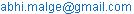(A. M Malge)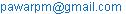(P. M Pawar)

Abhijeet M Malge, Prashant M Pawar. Analysis of Lift and Drag Forces at Different Azimuth Angle of Innovative Vertical Axis Wind Turbine. International Journal of Energy and Power Engineering .Special Issue: Energy Systems and Developments.Vol.4, No. 5-1, 2015, pp. 12-16. doi: 10.11648/j.ijepe.s.2015040501.12

Abstract:Vertical Axis Wind Turbines development was ignored as compared to horizontal axis wind turbines, due to its inability to generate large power. VAWT's have the advantage of working in turbulent wind and at low height. The power generated by VAWT depends upon the drag and lift forces acting on the blades. This paper is focused on analysis of drag and lift forces at different tip speed ratio acting at different azimuth angle of wind turbine. Computational fluid dynamics analysis of turbine is done by using K ω Shear Stress Transportation turbulence model. Computation is done to calculate Drag coefficients, Lift coefficients, and pressure and velocity distribution on wind turbine. Coefficient of lift is maximum at 35 o and minimum at 90 o, drag coefficient is maximum at 60o and minimum at 150o, pressure is maximum at 30 o and minimum at 90o.

Keywords:VAWT, CFD, Power Coefficient, Lift Force, Drag Force, Azimuth Angle

Contents

1. Introduction

VAWT has its pros and cons as compared to HAWT. VAWT do not require pitch and yawing, they can harness wind power coming from any direction. The gear box are installed on ground, due to which maintenance is easier. VAWT can be used at lower heights where the wind is turbulent which increases it’s utility in urban stand-alone power generation. However, it’s one of the drawback is high torque fluctuations with each revolution of blades. VAWT blades face inconsistent angle of attack through 360oof rotation. The lift and drag forces vary as the angle of attack varies. A detailed study should be done of the lift and drag forces generated

Earlier attempts were made to study the airfoil characteristics over 180 o  and 360o  angle of attack ,detailed aerodynamic flow around wind turbines airfoils has been carried in the region immediately before and after stall [1,2].Similar work on aerodynamic complexities of VAWT has been done using PIV techniques  .

Attempts were made to improve the self-starting speed of Darrius hydrokinetic wind turbine by studying the forces acting on the straight blades and varying the pitch angle . Novel vertical axis sail rotor was used and force analysis for one complete rotation was made for enhancement of self-starting speed .Effect of Cambered airfoil on self-starting speed of vertical axis wind turbine was studied and found that cambered airfoil improves the self-starting speed with low power coefficient as compared to symmetrical blades[6,7].

In this paper an innovative turbine were modelled in ANSYS.  Computational Fluid dynamics (Fluent) with K ω Shear Stress Transportation turbulence model has been used to simulate the lift and drag forces at different tip speed ratio and azimuth angle. Analysis of total lift and drag forces is made and its effect has been studied.

2. Numerical Analysis

ANSYS FLUENT has been used for complete analysis of wind turbine. Conservation of momentum in an inertial (non-accelerating) reference frame is described by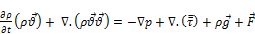(1)

The equation for conservation of mass, or continuity equation, can be written as follows: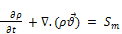(2)

ANSYS Fluent has been used for complete analysis of wind turbine. Using CFD simulation lift force, drag force and pressure on the turbine rotor is predicted. The 3 D geometry of Vertical Axis Wind Turbine has been created by using preprocessor Design Modular. Dimensions of the wind turbine are governed by wind tunnel test section size in which the experimentation is to be carried out. Turbine rotor diameter is 200 mm .NACA 68-613 cambered airfoil has been used in innovative VAWT. Cambered airfoil has the advantage of self-starting at low wind speed. Two blades placed orthogonal to each other with leading and trailing edge placed opposite to each other. Three flaps placed centrally between the two blades as shown in Figure.1.Flaps are made of 'S' shape so as to having concave and convex geometry.  The height of blades and flaps are 210 mm.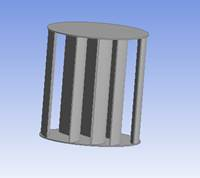Figure 1. Geometry of three story VAWT.

To perform the dynamic simulation, Moving Reference Frame (MRF) capability of CFD solver has been used which allows to model problems involving moving parts. The Moving reference frame is activated in selected zone, that modifies the equation of motion with additional acceleration terms which occurs due to transformation from stationary to Moving Reference Frame. By solving the equations in steady state manner, the flow around the moving part is modeled. The moving reference frame does not account for the relative motion of a moving zone with respect to adjacent zones. Thus the mesh remains fixed for computation. The turbine is enclosed in MRF coaxially. The MRF is enclosed in a computational flow domain. The flow domain is having cylindrical shape. It was extended in axial direction about 4 diameters upstream and 8 diameters downstream of the rotor. This was to make sure that air fully extended. The fluid flow domain diameter is twice the diameter of the rotor. The left side of the domain is named as velocity inlet. The blockage ratio is kept minimum to bring the simulation close to field testing.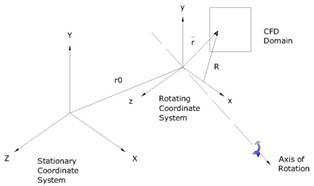Figure 2. MRF principle.

Meshing is unstructured with growth rate of 1.5.The skewness is 0.289 and the aspect ratio is 1.8.The total number of nodes is 1, 72,895.All the computations were done in ANSYS FLUENT. The solver used was Pressure based, absolute velocity with steady time. K-ω SST turbulence model with standard wall function is used. Cell zones created are turbine, MRF and Computational flow domain. Inlet velocity is varied in the range of 9- 11 m/sec. The pressure at the outlet is kept 0 Pascal. The turbine and MRF wall is considered as moving wall with rotational velocity along Z axis with no slip.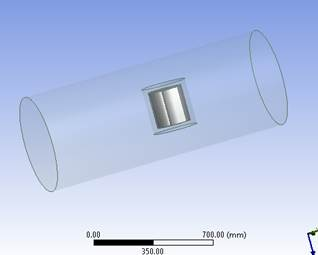Figure 3. Turbine model placed in Moving Reference Frame and Stationary Computational Domain.

3. Result and Discussion

Vertical axis wind turbine model having two blades and three flaps has been simulated for tip speed ratio range from 2.0-2.55.The rotational speed range of rotor is taken 900-1100 rpm. Lift force and drag force were noted for different azimuth angle ranging from 0-1800 .Maximum pressure, pressure and velocity distribution was also studied.

3.1. Tip Speed Ratio (TSR)

The TSR is calculated as the ratio between peripheral velocity and upstream wind velocity: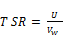Eq (3)

3.2. Lift Force and Drag Force

The forces driving Vertical Axis Wind Turbine can be described in more detail with the help of Figure 5. There are two important velocity components. There is the velocity of the airfoil relative to the shaft, which is at all times parallel to the chord, having a magnitude equal to the rotational speed multiplied by the radius. There is also the velocity of the wind, which is approximated as a constant velocity in one direction. The resultant of these two velocities is the velocity of the air relative to the airfoil. The angle between this resultant velocity and the chord of the airfoil is called the angle of attack (α).

Lift is created by a pressure differential, which occurs whenever there is an angle of attack, α, not equal to zero. In the 0° azimuth position and the 180° position, α = 0°. At this point, only a drag force exists. Lift begins to be created as the blades rotate out of these two positions and α increase .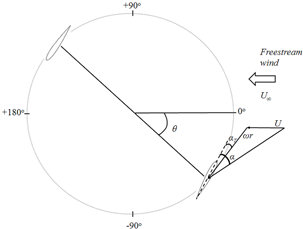Figure 5. Lift and srag forces acting on blade at different azimuth angle.

The lift and drag forces are found numerically. Coefficient of lift and drag are calulated by Eq(4) and Eq(5).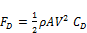(4)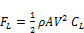(5)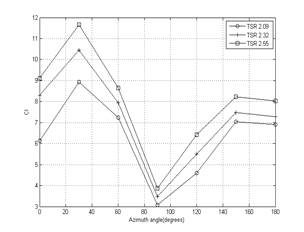Figure 6. Lift coefficient vs Azimuth angle.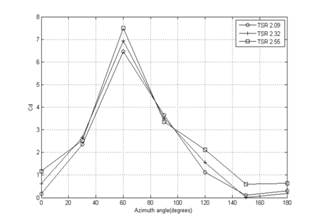Figure 7. Drag coefficent vs Azimuth angle.

Figure 6 shows lift behaviour of lift force with respect to azimuth angle at diffrent tip speed ratio. It is seen that lift force is maximum at 30oand then further reduces to minimum at 90 o  and then starts increasing gradually from 90oand reaches next peak to 150ofor the rotation of rotor  from 0-180.At 0o the lift force component is parallel to the wind flow where no tangential force is exerted on the blade. As the the blade rotates from 0-90othe lift force acting on the blade goes on increasing which in turn exerts tangential force on the blade . As the blades are fixed ,their movement is  constrained, that makes the turbine to rotate. As the blade  progresses from 90-180o , it is covered by flaps due to which the blade is  not exposed to wind . In this turbine two blades are used one of the blade is exposed to wind which  contribute to lift and generation of tangential force.From figure 7, it is found that drag force is maximum at 60 o and then reduces to minimum at 150o.The drag force on the turbine is because of the wind force acting on the concave and convex shape of the flaps. As the drag coefficient for concave surface is more than that of convex shape which dominate and rotates the turbine.

3.3. Static Pressure and Velocity Contours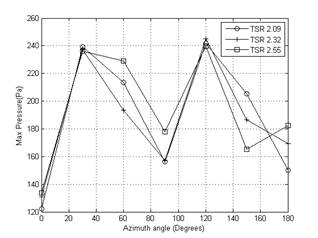Figure 8. Max Pressure vs Azimuth angle.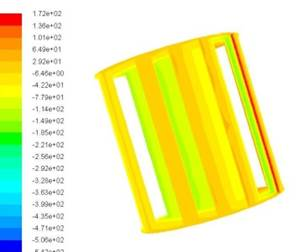(a)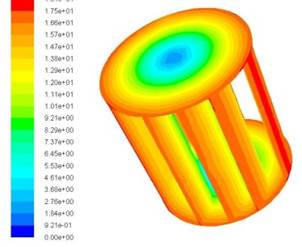(b)

Figure 9. Static Pressure and velocity contours.

From figure 8, it reveals that pressure magnitude escalates from 0-30o and then further diminishes to minimum at 90o , reoccurence of peak at 120ois seen again reducing to 150o.Figure 9 (a) shows static pressure plots. Pressure on the up stream side of the turbine is maximum as compared to the down stream side of the turbine. this pressure diffrence between upstream side and down stream side causes lift force in the turbine which makes it to rotate.Figure 9(b) shows velocity contour, in which it is seen that velocity aggravates from centre to its periphery.

4. Conclusion

A innovative vertical axis wind turbine has been modelled in design modeler of ANSYS and computational fluid dynamics analysis has been done in fluent. K ω Shear Stress Transportation tubulence model has been used. An attempt has been made to design the turbine in the view of harnessing maximum power in the wind.The coefficient of power developed by the turbine depends upon lift force, drag force  and pressure  acting on the turbine blades and flaps at diffrent azimuth positions at diffrenet tip speed ratio.Lift force is maximum at 30 o and then further reduces to minimum at 90 o  and then starts increasing gradually from 90o and reaches next peak to 150o for the rotation of rotor  from 0-180.At 0o the lift force component is parallel to the wind flow where no tangential force is exerted on the blade. As the the blade rotates from 0-90othe lift force acting on the blade goes on increasing which in turn exerts tangential force on the blade .

It is found that drag force is maximum at 60 o and then reduces to minimum at 150o.The drag force on the turbine is because of the wind force acting on the concave and convex shape of the flaps. As the drag coefficient for concave surface is more than that of convex shape which dominate and rotates the turbine.Pressure magnitude rises from 0-30o and then further diminishes to minimum at 90o , reoccurence of peak at 120ois seen again reduces to 150o.Pressure on the up stream side of the turbine is maximum as compared to the down stream side of the turbine. This pressure diffrence between upstream side and down stream side causes lift force in the turbine which makes it to rotate.Velocity aggravates from centre to its peripheryof the turbine.

5. Nomenclature

A: Swept Area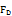: Drag force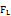: Lift force: Wind velocity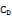: Coefficient of drag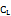: Coeffcient of lift

Sm: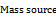: Force vector

U: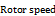: Density: Stress tensor

References

1. Haran,A. P., Soundranayagam,S.,2014," Experimental Charecteristics of wind turbine blading over full  0-360oangle of attack," Centre for wind Energy Technology, Department of Aeronautical Engineering, Park College of Engineering and Technology  Coimbatore.
2. Ferreira,S. C.J., Bijil,H., Bussel,G.V.,Kuik.G.V., 2007,"Simulating Dynamic Stall in a 2D VAWT Modeling statergy,verification and validation with Particla Image Velocimetry data," Journal of Physics,75,pp.1-14.
3. Sheldhal,R.E.,Kilmas,P.C.,1981,"Aerodynamic Charecteristics of Seven Symmetrical Airfoil Sections Through 180- Degree Angle of Attack for Use in Aerodynamic Analysis of Vertical Axis Wind Turbines. Sandia National Laboratories energy report".
4. Lazauskas, L., Kirke, B. K., 2012, Mode ling passive variable pitch cross ﬂow hydrokinetic turbines to maximize performance and smooth operation. Renewable Energy.45, pp. 41-50.
5. Dereng, V. G., 1981. Fixed geometry self-starting transverse axis wind turbine. United States patent 4264279.http://www.freepatentsonline.com/4264279.html.
6. Hurley, B., 1979,"A novel vertical axis sail rotor, "Wind Energy Workshop, 1st, Cranfield, Beds., England, April 19, 20.In  Proceedings. (A81-13851 03-44) London, Multi-Science Publishing Co., Ltd, pp.40-47.
7. Beri, H., Yao, Y., 2011,"Effect of cambered airfoil on self-starting of Vertical Axis Wind Turbine," Journal of Environmental Science and Technology,4 (3), pp. 302-312.
8. Amano, R. S., and Malloy, R.J. (2009), "CFD Analysis on Aerodynamic Design Optimization of Wind Turbine Rotor Blade," World Academy of Science, Engineering and Technology, pp.60.
9. Sabaeifard, P., and Razzahi, H. (2012). "Determination of Vertical Axis Wind Turbine Optimal Configuration through CFD Simulation," International Conference on Future Environment, pp.28.
10. Parvez, N., and Mokthar, W. (2012), "CFD Study of a Darreius Vertical Axis Wind Turbine," Proc ASEE North Central Section Conference.
11. Summer J., C. and Massonk, C. (2010), "CFD in Wind Energy: The Virtual Multiscale Wind Tunnel," Energies, 3, pp. 989-1013.
12. Dobrev, I., and Massouh .F.R. (2011), "CFD and PIV investigation of unsteady flow through Savonius wind turbine" Energy Procedia, 6, pp. 711-720.
13. Rajkumar, S., Ravindran, D.(2010), "Computation Fluid Dynamics at various Angle of Attack and Low Reynolds number." International Journal of Engineering Science and Tech.
14. Howell, R., Qin, N., Edward, J., Durrani, N., (2010), "Wind Tunnel and Numerical Study of a small Vertical Axis Wind Turbine," Renewable Energy, 35, pp.412-422.
15. Yao, J., Jianliang, W., Yuan W., Wang, H., Cao, L. (2012). "Analysis on the influence of Turbulence model changes to aerodynamic performance of vertical axis wind turbine." International Conference on Advances in Computational Modelling and Simulation, Procedia Engineering, 31, 274 – 281.

 Contents 1. 2. 3. 3.1. 3.2. 3.3. 4. 5.
Article ToolsAbstractPDF(387K)